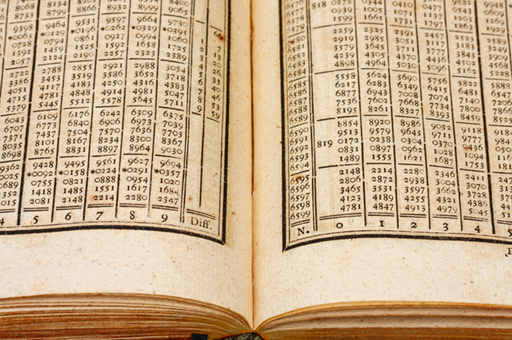Science, Maths & Technology

### Become an OU studentMathematics for science and technology

Start this free course now. Just create an account and sign in. Enrol and complete the course for a free statement of participation or digital badge if available.

# 1 Logarithms to base 10

Logarithms are very closely related to powers and can have any base number. However, one of the most commonly used was the logarithm to base 10, also known as the common logarithm. The process of taking a log to base 10, is the inverse (opposite operation) of raising the base 10 to a power.

In the example 103 = 1000, 3 is the index or the power to which the number 10 is raised to give 1000.

When you take the logarithm, to base 10, of 1000 the answer is 3.

This is written as:

Log10 (1000) = 3

Hence, the logarithm is the index to which the base was raised. Or: log10 10n = n

So, 103 = 1000 and log10 (1000) = 3 express the same fact but the latter is in the language of logarithms.

This idea can be generally defined as follows:

If a number y can be written in the form ax, then the index x is called the logarithm of y to the base a.

If y = ax

Then loga= x

Here are some more examples that provide some general logarithm rules.

Since 2 = 21, then log2 (2) = 1

Similarly, log3 (3) = 1, and log10 (10) = 1, and as a = a1, then loga (a) = 1

Also, since 1 = 20 then log2 (1) = 0

Similarly, log3 (1) = 0 and log10 (1) = 0, and as 1 = a0, then loga (1) = 0.

See how you get on in this activity.

## Activity 1 Taking the log to base 10

Without the use of a calculator, work out the values of:

1. log10 (10)
2. log10 (100)
3. log10 (0.01)
4. log10 (1 000 000)

1. logaa = 1

So, log10 (10) = 1

2. log10 (100)

10 raised to the power of what equals 100?

102 = 100

So, log10 (100) = 2

3. log10 (0.01)

10 raised to the power of what equals 0.01?

10–1 = 0.1 and 10–2 = 0.01

So, log10 (0.01) = –2

4. log10 (1 000 000) = 6

Since, 106 = 1 000 000

This activity and preceding section allows the following basic observations about logs to be made.

The log of anything < 1 is a negative number.

The log of anything > 1 is a positive number.

The log of 1 is zero to any base.

Before the days of the electronic calculator, logarithms were used everyday for multiplication and division and involved the use of log tables, as shown in Figure 1.Figure 1 200 year old log tables

Nowadays, logarithms are mainly used in calculus or to find a linear function from an exponential one. You’ll be glad to hear that you don’t need a book of log tables now to work with logarithms.

On your calculator you should have a key called log (with the inverse function as 10x or similar). This key allows you to take the log in base 10 of any positive number.

Try now in this quick activity.

## Activity 2 Using a calculator to take logs

Use your calculator to take the log to base 10 of the following, showing your answers to 4 decimal places:

1. log10 (25)

2. log10 (1.5)

3. log10 (2500)

4. log10 (0.0032)